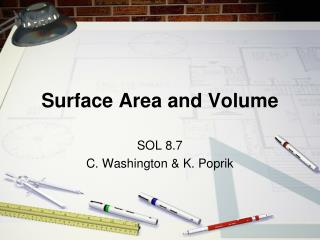DownloadDownload PresentationSurface Area and Volume

# Surface Area and Volume

Télécharger la présentation## Surface Area and Volume

- - - - - - - - - - - - - - - - - - - - - - - - - - - E N D - - - - - - - - - - - - - - - - - - - - - - - - - - -
##### Presentation Transcript

1. Surface Area and Volume SOL 8.7 C. Washington & K. Poprik

2. What is a rectangular prism? • TV • Locker • Gift Box Cereal Box Height Width Length

3. What is a cylinder? • Pringles • Container • Toilet Paper • Roll Soup Can Radius Made up of 2 circles & one rectangle Height

4. Surface Area The sum of all the areas of all the shapes that make up an object The label on a can The wrapping paper on a present The amount of material needed to make a can or box

5. Surface Area L W 2 2lw

6. Surface Area L H 2 2lh

7. Surface Area W H 2 2wh

8. Surface Area 2lw 2lh 2wh + + TO SOLVE: Remember to square the units on your answer! Plug in all #s Multiply Add

9. Let’s Try One Together Plug in all #s Multiply Add

10. Try One Alone 15in 2in 8in Plug in all #s Multiply Add

11. Surface Area height Length (circumference of the circle )

12. Surface Area + TO SOLVE: Remember to square the units on your answer! Plug in all #s Multiply Add

13. Let’s Try One Together Plug in all #s Cancel out exponent then, Multiply Add

14. Try One Alone 5in 20in Plug in all #s Cancel out exponent then, Multiply Add

15. Try It with a Partner Complete #s: 8, 10, 13, & 14 on the practice sheet on the back of your notes!

16. Volume The number of cubic units needed to fill the space occupied by a solid. The amount of soup that could fit in a can The amount of Pringles that can fit in the container The amount of cereal that can fit in the cereal box

17. Volume Remember to cube the units on your answer! TO SOLVE: Plug in all #s Multiply Straight Across

18. Let’s Try One Together Plug in all #s Multiply

19. Remember to cube the units on your answer! Volume TO SOLVE: Plug in all #s Get rid of exponent Multiply Straight Across

20. Let’s Try One Together Plug in all #s Cancel out exponent Multiply